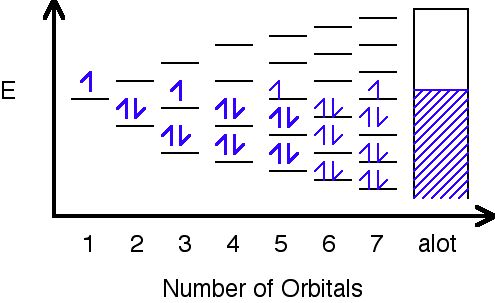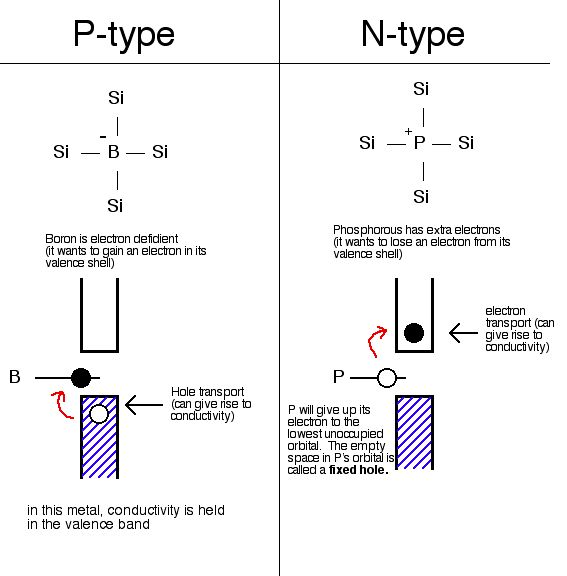# 6.8D: Band Theory of Semiconductors

Band Theory was developed with some help from the knowledge gained during the quantum revolution in science. In 1928, Felix Bloch had the idea to take the quantum theory and apply it to solids. In 1927, Walter Heitler and Fritz London discovered bands- very closely spaced orbitals with not much difference in energy.In this image, orbitals are represented by the black horizontal lines, and they are being filled with an increasing number of electrons as their amount increases. Eventually, as more orbitals are added, the space in between them decreases to hardly anything, and as a result, a band is formed where the orbitals have been filled.

Different metals will produce different combinations of filled and half filled bands.Sodium's bands are shown with the rectangles. Filled bands are colored in blue. As you can see, bands may overlap each other (the bands are shown askew to be able to tell the difference between different bands). The lowest unoccupied band is called the conduction band, and the highest occupied band is called the valence band.

Bands will follow a trend as you go across a period:

• In Na, the 3s band is 1/2 full.
• In Mg, the 3s band is full.
• In Al, the 3s band is full and the 3p ban is 1/2 full... and so on.

The probability of finding an electron in the conduction band is shown by the equation:

$P= \dfrac{1}{e^{ \Delta E/RT}+1}$

The ∆E in the equation stands for the change in energy or energy gap. t stands for the temperature, and R is a bonding constant. That equation and this table below show how the bigger difference in energy is, or gap, between the valence band and the conduction band, the less likely electrons are to be found in the conduction band. This is because they cannot be excited enough to make the jump up to the conduction band.

ELEMENT ∆E(kJ/mol) of energy gap # of electrons/cm^3 in conduction band insulator, or conductor?
C (diamond) 524 (big band gap) 10-27 insulator
Si 117 (smaller band gap, but not a full conductor) 109 semiconductor
Ge 66 (smaller band gap, but still not a full conductor) 1013 semiconductor

## Conductors, Insulators and Semiconductors### A. Conductors

Metals are conductors. There is no band gap between their valence and conduction bands, since they overlap. There is a continuous availability of electrons in these closely spaced orbitals.

### B. Insulators

In insulators, the band gap between the valence band the the conduction band is so large that electrons cannot make the energy jump from the valence band to the conduction band.

### C. Semiconductors

Semiconductors have a small energy gap between the valence band and the conduction band. Electrons can make the jump up to the conduction band, but not with the same ease as they do in conductors.

There are two different kinds of semiconductors: intrinsic and extrinsic.

#### i. Intrinsic Semiconductors

An intrinsic semiconductor is a semiconductor in its pure state. For every electron that jumps into the conduction band, the missing electron will generate a hole that can move freely in the valence band. The number of holes will equal the number of electrons that have jumped.#### ii. Extrinsic Semiconductors

In extrinsic semiconductors, the band gap is controlled by purposefully adding small impurities to the material. This process is called doping. Doping, or adding impurities to the lattice can change the electrical conductivity of the lattice and therefore vary the efficiency of the semiconductor. In extrinsic semiconductors, the number of holes will not equal the number of electrons jumped. There are two different kinds of extrinsic semiconductors, p-type (positive charge doped) and n-type (negative charge doped).## Problems

1. How do you distinguish between a valence band and a conduction band?
2. Is the energy gap between an insulator smaller or larger than the energy gap between a semiconductor?
3. What two methods bring conductivity to semiconductors?
4. You are more likely to find electrons in a conduction band if the energy gap is smaller/larger? 5. The property of being able to be drawn into a wire is called...